Contents

cohomology

# Contents

## Idea

A graph complex is a certain cochain complex spanned by equivalence classes of certain labeled directed graphs, whose differential encodes the operation of contracting away edges in a graph.

Two similar but different classes of examples are usually referred to by default as just “the graph complex”, going back to hints in Kontsevich 92, P. 11-12, Kontsevich 93, 5:

Given a smooth manifold $\Sigma$, often taken to be Euclidean space $\mathbb{R}^D$, there is

1. a graph complex model for the real cohomology of the unlabeled ordered configuration space of points in $\Sigma$

2. a graph complex model for the real cohomology of the space of knots in $\Sigma$.

In both cases, the graphs are interpreted as Feynman diagrams for Chern-Simons theory and the map which identifies these with cocycles in real cohomology of either the (unlabeled & ordered) configuration space of points or the space of knots is given by sending a Feynman diagram to its Feynman amplitude. In the first case this is an n-point function, regarded as a differential form on the configuration space of $n$-points, while in the second case this is a vacuum amplitude depending on the isotopy class of the Wilson loop encoded by the knot – a Vassiliev knot invariant.

Beware that these graph complex models differ only in very small technical detail as the manifold $\Sigma$ varies and the choice between models for configuration space of points or spaces of knots is made (see the Overview of definitions below), and yet these small details completely change the cohomological nature of the resulting graph complexes; a point not often made manifest when any given author discusses “the graph complex”.

### Model for ordered configuration spaces of points

The graph complex model for unlabeled & ordered configuration spaces of points was originally sketched in Kontsevich 92 (p. 11-12) and worked out in detail in Lambrechts-Volić 14 for $\Sigma = \mathbb{R}^D$ a Euclidean space. Other authors have claimed generalization to $\Sigma$ a closed manifold (Campos-Willwacher 16) possibly with boundary (Campos-Idrissi-Lambrechts-Willwacher 18).

Here we denote this version of the graph complex by “$Graphs$”, in contrast to “$KnotGraphs$” for the other model, discussed further below.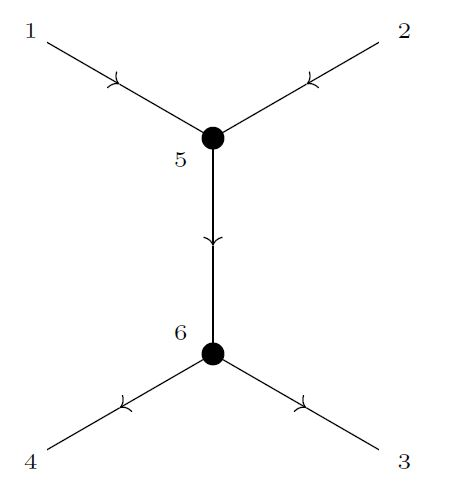This graph complex is spanned by finite directed graphs with linearly ordered internal and external vertices, subject to a sign rule reflecting the orientation of edges. The differential sends any graph to a signed sum of graphs obtained by contracting one of the edges with at least one internal vertex.

Under ordered disjoint union of edges and internal vertices this cochain complex becomes a differential graded-commutative algebra

$Graphs_n\big( \mathbb{R}^D \big) \;\in\; dgcAlg_{\mathbb{R}} \phantom{AA} n, D \in \mathbb{N} \,,$

As such, this is quasi-isomorphic to the semi-algebraic de Rham algebra

$\Omega^\bullet \big( \underset{{}^{1,\cdots,n}}{Conf} \big( \Sigma \big) \big) \;\in\; dgcAlg_{\mathbb{R}} \phantom{AA} n, D \in \mathbb{N}$

of the Fulton-MacPherson compactification of the configuration space of points $\underset{{}^{1,\cdots,n}}{Conf}(\mathbb{R}^D)$ for $n$ points in $D$-dimensional Euclidean space.

The chain map which exhibits this quasi-isomorphism is given by regarding a graph as a Feynman diagram for Chern-Simons theory on $\Sigma = \mathbb{R}^D$ and sending it to its corresponding Feynman amplitude, namely to the configuration space-integral of the wedge product of Chern-Simons propagators associated to the edges, regarding Feynman amplitudes as differential forms on configuration spaces of points:

(1)$\underset{ \color{blue} \array{ \phantom{A} \\ \text{graph complex} \\ \text{of n-point Feynman diagrams} \\ \text{for Chern-Simons theory} \\ \text{on} \; \Sigma } }{ Graphs_n(\Sigma) } \underoverset{ \simeq_{\mathrlap{qi}} } { \color{blue} \array{ \text{assign Feynman amplitudes} \\ \text{of Chern-Simons theory} \\ \phantom{A} } } { \longrightarrow } \underset{ \color{blue} \array{ \phantom{A} \\ \text{de Rham algebra of} \\ \text{ordered configuration space} \\ \text{of n points in}\; \Sigma } }{ \Omega^\bullet \big( \underset{{}^{ \{1,\cdots,n \} }}{Conf}\big( \Sigma \big) \big) } \,.$

This means that for each edge in a graph a Chern-Simons propagator is assigned, and for each of $n_{int} \in \mathbb{N}$ internal vertices the wedge product of its adjacent Chern-Simons propagators is fiber integrated along the canonical fibration of configuration spaces of points:

$\underset{ {}^{\{1, \cdots, n + n_{int} \}} }{ Conf } \big( \mathbb{R}^D \big) \longrightarrow \underset{ {}^{\{1,\cdots,n\}} }{ Conf } \big( \mathbb{R}^D \big)$

just as befits the definition of a Feynman amplitude when regarding them as differential forms on configuration spaces of points.

### Model for spaces of knots

The graph complex model for spaces of knots was originally sketched in Kontsevich 93, Section 5 and worked out in Cattaneo, Cotta-Ramusino, Longoni 02, 05, see also Bar-Natan 91.

Here we denote this version of the graph complex by “$KnotGraphs$”, in contrast to “$Graphs$” for the other model, discussed above.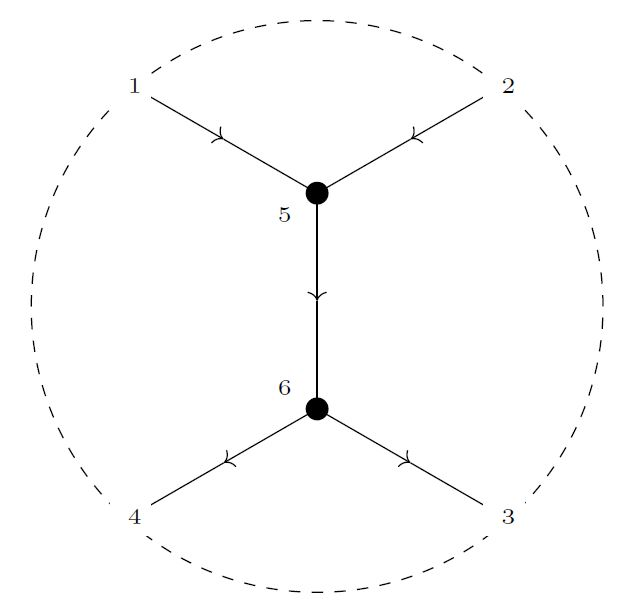The explicit definition is almost exactly the same as that of the model for configuration spaces of points above, except that the external vertices here have a degree -1 instead of 0, and that the differential sees contractible edges between consecutive external vertices, often called arcs, shown by dashed lines on the right.

Together these two innocent modifications make the graphs now represent vacuum Feynman diagrams for Chern-Simons theory in the presence of a Wilson loop, with the external vertices now attached to this knot (corresponding to the dashed line shown on the right):

(2)$\underset{ \color{blue} \array{ \phantom{A} \\ \text{graph complex} \\ \text{of n-point Feynman diagrams} \\ \text{for Chern-Simons theory} \\ \text{on} \; \Sigma \\ \text{in the presence of a Wilson loop knot} } }{ KnotGraphs_n(\Sigma) } \underoverset{ } { \color{blue} \array{ \text{assign Feynman amplitudes} \\ \text{of Chern-Simons theory} \\ \phantom{A} } } { \longrightarrow } \underset{ \color{blue} \array{ \phantom{A} \\ \text{de Rham algebra} \\ \text{of the space of knots} \\ \text{in}\; \Sigma \\ \text{(higher Vassiliev knot invariants)} } }{ \Omega^\bullet \big( Emb(S^1, \Sigma \big) \big) } \,.$

This yields higher Vassiliev knot invariants, a good review is in Volić 13. It is a conjecture (Volić 13, Conjecture 4.8) that this map, too, is a quasi-isomorphism.

### Model for other spaces

There are yet other, inequivalent, graph complexes. Notably there is a type of graph complex whose (co)homology in degree 0 is the Lie algebra of the Grothendieck-Teichmüller group (Willwacher 10, Dolgushev-Rogers 12), and this is neither of the above two cases. (…)

## Definition

### Overview

There are two different classes of (Kontsevich-) graph complexes, modelling the real cohomology of, respectively,

configuration spacegraph complex
configuration spaces of points$\underset{{}^{1,\cdots,n}}{Conf}(\Sigma)$$\;\;\leftrightarrow\;\;$$Graphs_n(\Sigma)$(1)
spaces of knots$Emb(S^1,\Sigma)$$\;\;\leftrightarrow\;\;$$KnotGraphs(\Sigma)$(2)

Despite their very deifferent cohomological nature, the explicit definitions of these two kinds of graph complexes are mostly identical, except for some small but crucial difference in the definition of degrees and labels of edges (see below).

In addition, the definition of the graph complex model for configuration spaces of points depends on a choice of smooth manifold $\Sigma$, possibly with boundary, namely such that the graph complex $Graphs(\Sigma)$ provides a model for the cohomology of the configuration space of points in $\Sigma$

$Graphs\big( \Sigma \big) \leftrightarrow \Omega^\bullet\big( Conf(\Sigma) \big) \,.$

The configuration spaces of points are naturally filtered by subspaces of a fixed number $n \in \mathbb{N}$ of points. In the graph complexes this correpsonds to graphs with a fixed number $n$ of external vertices:

$\underset{ \mathclap{ \color{blue} \array{ \phantom{A} \\ \text{graphs with} \\ n\;\text{external vertices} } } }{ Graphs_n\big( \Sigma\big) } \;\;\;\leftrightarrow\;\;\; \Omega^\bullet\big( \underset{ \mathclap{ \color{blue} \array{ \phantom{A} \\ \text{configuration space} \\ \text{of}\;n\;\text{points} } } }{ \underbrace{ \underset{{}^{1,\cdots,n}}{Conf}(\Sigma) } } \big) \,.$

The full graph complexes/configuration spaces are just the direct sum/union of those for fixed number $n$ of external vertices/points, hence one may focus attention on the latter.

The precise form of these relations is the content of the theorems discussed below. Before stating this in detail, we make some general remarks on how the situation depends on $\Sigma$:

Beware that the graphs $[\Gamma] \in Graphs_n(\Sigma)$ themselves are not going to carry an embedding into the manifold $\Sigma$, they are just abstract graphs. But the construction of the above correspondence to the cohomology of the configuration space of points is given by associating with a graph the corresponding correlator in a Chern-Simons perturbative quantum field theory on the space $\Sigma$.

As a consequence, the dependence of the graph complexes themselves on $\Sigma$ is mild:

In particular, in the case that $\Sigma = \mathbb{R}^D$ is a Euclidean space, the corresponding graph complexes $Graphs\big( \mathbb{R}^D \big)$ depend essentially only on whether the dimension $D$ is even or odd. Concretely, the degree of a graph in the graded vector space $Graphs_n(\mathbb{R}^D)$ is

(3)$deg\big( [\Gamma]\big) \;=\; (D-1) \cdot \#\!Edges - D \cdot \#\!Vertices_{int} \,,$

hence each edge contributes a degree $D-1$ and each internal vertex a degree $-D$. As a consequence, due to the sign rule in the graded-commutative algebra structure on $Graphs_n(\mathbb{R}^D)$ we have the following parities

$\Sigma = \mathbb{R}^D$edgesinternal vertices
$D$ evenoddeven
$D$ oddevenodd

Up to an absolute even change of grading, the graph complexes $Graphs_n(\mathbb{R}^D)$ depend only on these parities, hence depend only on whether $D$ is even or odd.

Finally, beware that many authors consider the case where $\Sigma = \mathbb{R}^D$ by default, and don’t mention a dependence on a choice of manifold, but just on a natural number $D$. This natural number is then often denoted “$d$” or “$n$”.

For instance:

• the “graph complex with anti-symmetric set of edges” of Bar-Natan & McKay, Def. 3.3 is denoted $GC_2$ in Willwacher 10 and is our $Graphs_0\big(\mathbb{R}^2\big)$;

• similarly $GC_3$ in Koroshkin-Willwacher-Živković 14 is our $KnotGraphs\big( \mathbb{R}^3\big)$.

(While the cohomology of $Graphs_0(\Sigma)$ – being equivalent to that of $Conf_0(\Sigma) \simeq \ast$ – is trivial, these authors consider further filtrations which have non-trivial cohomology in filtration stages, see Bar-Natan & McKay, Def. 3.6).

### 1) Graph complexes modelling configuration spaces of points

We discuss graph complexes $Graphs_n(\Sigma)$ modelling the real cohomology of configuration spaces of points $\underset{{}^{1,\cdots,n}}{Conf}(\Sigma)$.

For the case of $\Sigma = \mathbb{R}^D$ these were originally hinted at in Kontsevich 92 (p. 11-12) and discussed in detail in Lambrechts-Volić 14.

#### $Graphs_n\big( \mathbb{R}^3 \big)$

We state the definition of the graph complexes $Graphs_n\big( \mathbb{R}^3\big)$ (as motivated above, see Def. below) associated with Euclidean space of dimension 3, following Lambrechts-Volić 14, Section 6.

##### Graphs
###### Definition

(graphs)

In the following, by a graph we mean specifically:

1. $Edg \underoverset{t}{s}{\rightrightarrows} Vert \,,$
2. a decomposition of the finite set $Vert$ of vertices as a disjoint union

$Vert \simeq Vert_{ext} \sqcup Vert_{int}$

of “external” and “internal” vertices.

3. a linear order on $Vert$, such that $Vert_{ext} \lt Vert_{int}$.

Hence both $Vert_{ext}$ and $Vert_{int}$ are linear orders, and

$Vert = Vert_{ext} \sqcup_{{}_{\lt}} Vert_{int}$

is their ordered disjoint union.

An isomorphism $\Gamma \simeq \Gamma'$ of such graphs is a pair of bijections between sets of edges and vertices

(4)$\array{ Edg &\underoverset{t}{s}{\rightrightarrows}& Vert_{ext} \sqcup Vert_{int} \\ \mathllap{{}^{\simeq}}\big\downarrow && \mathllap{{}^{\simeq}}\big\downarrow \\ Edg' &\underoverset{t'}{s'}{\rightrightarrows}& Vert'_{ext} \sqcup Vert'_{int} }$

which respects all structure, hence the source and target maps, the decomposition into external and internal vertices, and the linear order.

###### Remark

(empty graph)

The empty graph counts as a graph, according to Def. .

We write $\#\! Vert \coloneqq \left\vert Vert \right\vert$ for the cardinality of the sets of vertices, etc.

###### Remark

(denotation for linear order on vertices)

The linear order on the vertices in Def. is equivalently a choice of bijection of $Vert$ with the set of the first $\#\!Vert$ natural numbers

(5)$Vert \;\simeq\; \big\{ 1, 2, \ldots, \# Vert_{ext}, \# Vert_{ext} + 1, \ldots , \# Vert_{ext} + \# Vert_{int} \big\}$

With this understood, we may depict graphs as as diagrams with oriented edges and numbered vertices.

###### Definition

(degree of a graph)

Given a graph

$\Gamma \;=\; \big( Edg \underoverset{t}{s}{\rightrightarrows} Vert_{ext} \sqcup Vert_{int} \big)$

according to Def. , its degree is the natural number

$deg(\Gamma) \;\coloneqq\; 2 \cdot \#\!Edg - 3 \cdot \#\!Vert_{int} \,,$

hence the sum of 2 for each edge and of -3 for each internal vertex.

If we also agree to

1. denote internal vertices by a bullet;

2. denote external vertices by… no denotation (as usual for Feynman diagrams!)

then the ingredients of these graphs are as shown in the following figure: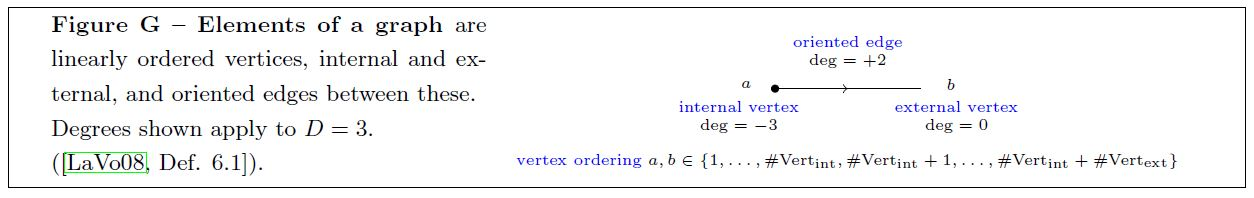The following shows some very simple examples of graphs, depicted this way: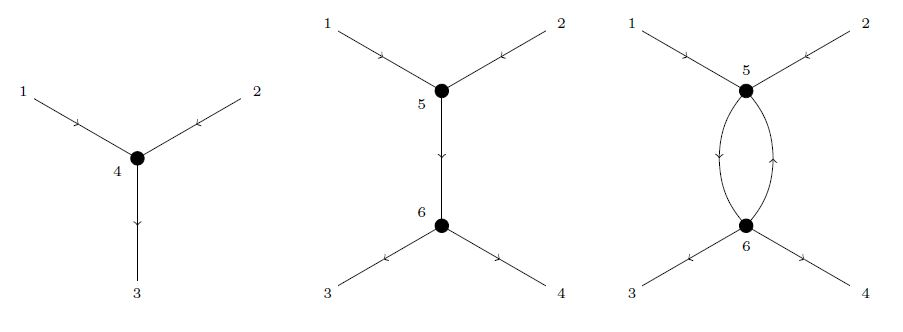Beware that graphs need not be planar, may have edges between two external vertices (chords) and other effects not seen in these simple examples (… need to draw more generic examples…).

In particular, graphs may have tadpoles. But the next definition makes such graphs vanish in the graph complex:

###### Definition

(sign rules for graphs)

For a fixed finite linear order $Vert_{ext} \simeq \{1, 2, \cdots, \#\!Vert_{ext}\}$, consider the real linear span on the set of isomorphism classes (4) of graphs, according to Def. , with that set of external vertices:

(6)$\mathbb{R} \Big[ \big\{ Edg \underoverset{t}{s}{\rightrightarrows} Vert_{ext} \sqcup Vert_{int} \;\vert\; Vert_{int} \in FinLinOrd \big\}_{/\sim} \Big] \;\in\; Vect_{\mathbb{R}} \,.$

The degree associated with a graph (Def. ) makes this a graded vector space.

On this real vector space, consider the linear equivalence relation $\sim$ generated by

(7)$\Gamma \;\sim\; -\Gamma' \phantom{AA} \array{ \text{if}\;\Gamma'\; \text{is}\;\Gamma\;\text{with} \\ \text{orientation of one edge reversed} }$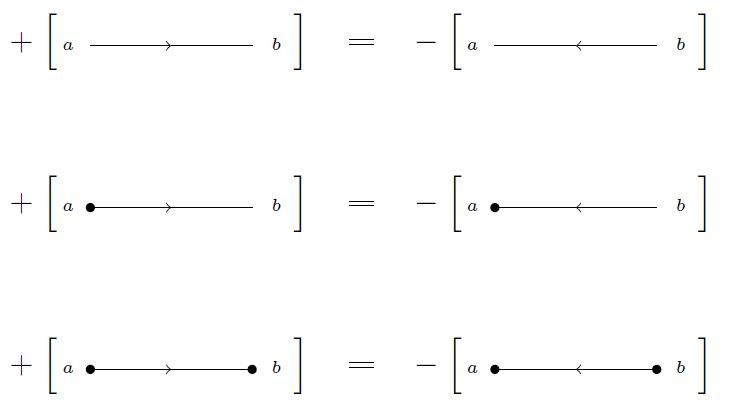and

(8)$\Gamma \;\sim\; -\Gamma' \phantom{AA} \array{ \text{if}\;\Gamma'\;\text{is}\;\Gamma\;\text{with} \\ \text{a pair of consecutive internal vertices transposed} }$

Since this equivalence relation is linear, the set of its equivalence classes is still a vector space, and since the equivalence relation also preserves the degrees (Def. ), it is still a graded vector space:

###### Definition

For $n \in \mathbb{N}$, $n \coloneqq \#\! Vert_{ext}$, we write

(9)$\widehat{Graphs}_n\big(\mathbb{R}^3\big) \;\coloneqq\; \mathbb{R} \Big[ \big\{ Edg \underoverset{s}{t}{\rightrightarrows} Vert_{ext} \sqcup Vert_{int} \;\vert\; Vert_{int} \in FinLinOrd \big\}_{/\sim} \Big]_{/\sim} \;\in\; Vect_{\mathbb{R}} \,.$

for the real graded vector space of equivalence classes under the sign rules (7) and (8) in the linear span (6) of the isomorphism classes of graphs (Def. ) with $n$ external vertices.

For $\Gamma$ any graph, we write

(10)$\big[ \Gamma \big] \;\in\; \widehat{Graphs}_n\big(\mathbb{R}^3\big) \,.$

for the equivalence class of the element in this real graded vector space that it represents.

When there is not risk of confusion, we will still refer to this equivalence class $[\Gamma]$ as a graph.

A further quotient space $Graphs_n(\mathbb{R}^3)$ of $\widehat Graphs_n(\mathbb{R}^3)$ (9) will underly the actual graph complex below.

###### Example

A direct consequence of quotienting out the equivalence relation (7) is that graphs with “tadpoles”, namely with edges that have coinciding source and target vertex, vanish in $\widehat Graphs_n(\mathbb{R}^3)$ (9):

$e \in Edg_\Gamma \;\text{s.t.}\; s(e) = t(e) \phantom{AA} \Rightarrow \phantom{AA} \big[ \Gamma \big] \;=\; 0$

(This holds more generally for all $\mathbb{R}^D$ with odd $D = 2k + 1$.)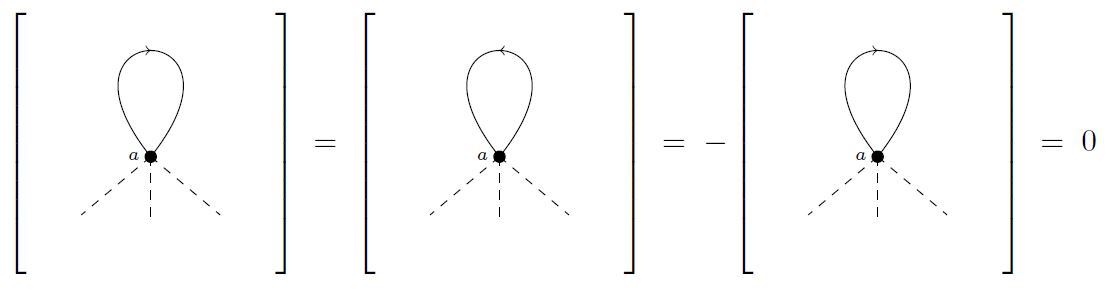###### Example

(trivalent graphs)

A graph without external vertices has degree (Def. ) equal to zero precisely if all its vertices (which are all internal, by assumption) are trivalent:

$\#\!Verts_{ext} = 0 \;\;\Rightarrow\;\; \big( deg(\Gamma) = 0 \;\;\Leftrightarrow\;\; \text{all vertices are trivalent} \big) \,.$

The following is the smallest example of such a trivalent graph: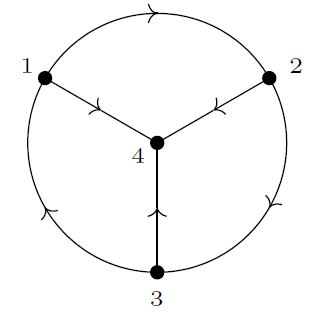Hence in general, the degree of a trivalent graph is three times its number of external vertices:

$\Gamma \; \text{trivalent} \;\;\;\Rightarrow \;\;\; deg\big( [\Gamma] \big) \;=\; 3 \cdot \#\!Verts_{ext} \,.$

(Because such a graph is the result of taking a trivalent graph in degree 0 and replacing internal vertices of degree -3 with external vertices of degree 0.)

For example, the following graph, obtained from the above trivalent graph by replacing the three internal vertices labeled 1,2 and 3 with external vertices, is of degree $3 \cdot 3 = 9$: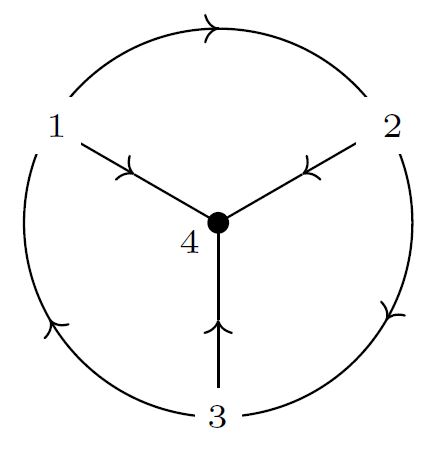##### The algebra of graphs
###### Definition

(wedge product of graphs)

For

$[\Gamma_1], [\Gamma_2] \;\in\; \widehat Graphs_n\big( \mathbb{R}^D\big)$

two equivalence classes (9) of isomorphism classes of graphs (Def. ) with the same number of external, their wedge product is the element

$[\Gamma_1] \wedge [\Gamma_2] \coloneqq [\Gamma_1 \wedge \Gamma_2] \;\in\; \widehat Graphs_n\big( \mathbb{R}^D\big)$

represented by the graph (Def. ) which is given by the disjoint union of linear orders of edges and internal vertices

$\Gamma_1 \wedge \Gamma_2 \;\coloneqq\; \Big( Edgs_{\Gamma_1} \sqcup_{{}_{\lt}} Edgs_{\Gamma_2} \underoverset{t_1 \sqcup_{\lt} t_2}{s_1 \sqcup_{\lt} s_2}{\rightrightarrows} Vert_{ext} \sqcup_{{}_{\lt}} \big( Vert_{int,1} \sqcup_{{}_{\lt}} Vert_{int,2} \big) \Big) \,.$

It is immediate that:

###### Lemma

For $n \in \mathbb{N}$, the graded vector space $\widehat Graphs_n\big( \mathbb{R}^3\big)$ (9) of graphs (Def. ) equipped with the wedge product of graphs from Def.

$\array{ \widehat{Graphs}_n(\mathbb{R}^3) \otimes \widehat{Graphs}_n(\mathbb{R}^3) &\overset{\wedge}{\longrightarrow}& \widehat{Graphs}_n(\mathbb{R}^3) \\ \big( [\Gamma_1], [\Gamma_2] \big) &\mapsto& [\Gamma_1 \wedge \Gamma_2] }$

##### The differential on graphs

The differential on the graded vector space of equivalence classes of graphs (9) is defined in terms of contraction of certain edges in the graph (Def. below). For this we first say which edges count as contractible (Def. ) and which sign is picked up when contracting them (Def. ):

###### Definition

(contractible edges)

Given a graph $\Gamma$ (Def. ) we say that an edge $e \in Edg_\Gamma$ is a contractible edge if the following conditions hold:

1. $e$ is not a tadpole (Example ):

$s(e) \neq t(e)$
2. $e$ has at least one internal vertex:

$s(e) \in Vert_{int} \phantom{AA} \text{or} \phantom{AA} t(e) \in Vert_{int}$
3. every internal vertex of $e$ is connected to more than just one other vertex, i.e. $e$ is not a solid arrow in a diagram of the following form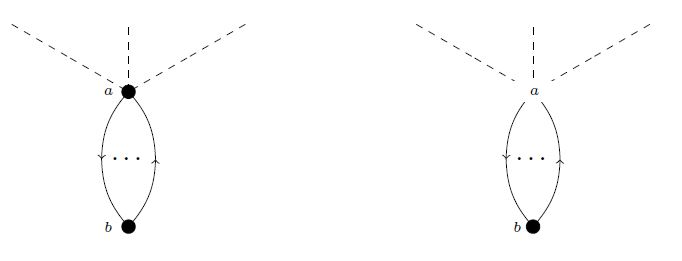We write

(11)$Edg^{contr}_\Gamma \;\subset\; Egd_\Gamma$

for the subset of the contractible edges inside all edges of a graph $\Gamma$.

###### Definition

(contraction of an edge)

Given a graph $\Gamma$ (Def. ) and a contractible edge $e \in Edg_\Gamma$ (Def. ) consider the induced graph obtained by removing that edge and the one of its vertices with the larger label

$\Gamma / e \;\coloneqq\; \big( Edg_\Gamma \setminus \{e\} \big) \underoverset{q \circ t}{q \circ s}{\rightrightarrows} \big( Vert_\Gamma \setminus \{ max(s(e),t(e)) \} \big)$

and connecting all edges previously attached with the removed vertex to the remaining vertex of that edge (ie. the one with the smaller label):

$\array{ Vert_\Gamma &\overset{}{\longrightarrow}& Vert_{\Gamma/e} \\ v &\mapsto& \left\{ \array{ min(s(e),t(e)) &\vert& \text{if}\; v = max(s(e),t(e)) \\ v &\vert& \text{otherwise} } \right. }$
###### Remark

(sign of a contractible edge)

Given a contractible edge $e \in Egd_\Gamma$ (Def. ) in some graph $\Gamma$ (Def. ) we associate a sign as follows:

$\epsilon(e) \;\coloneqq\; \left\{ \array{ (-1)^{ t(e) } &\vert& t(e) \gt s(e) \\ -(-1)^{s(e)} &\vert& s(e) \gt t(e) } \right. \,,$

where on the right we are identifying the linear order on the vertices with their natural number-labels as in (5).

###### Definition

(differential on graphs)

For $Vert_{ext}$ a fixed finite linear order of $n = \#\! Vert_{ext}$ external vertices, define on the graded vector space $\widehat Graphs_n\big( \mathbb{R}^3\big)$ (9) a linear endomorphism $d$ given on the class $[\Gamma]$ (10) of a graph $\Gamma$ (Def. ) by the linear combination of all its edge contractions $\Gamma/e$ (Def. ) for all contractible edges (Def. ) weighted by their sign (Def. ):

$\array{ \widehat Graphs_n\big( \mathbb{R}^3\big) &\overset{d}{\longrightarrow}& \widehat Graphs_n\big( \mathbb{R}^3\big) \\ [\Gamma] &\mapsto& \underset{ e \in Edg^{contr}_\Gamma }{\sum} \epsilon(\Gamma) \cdot [\Gamma/e] } \,.$
###### Example

(differential of propagator graph vanishes)

The differential from Def. vanishes on all graphs without any internal vertices (since these have no contractible edges in the sense of Def. ). In particular it vanishes on the graph for the single Chern-Simons propagator: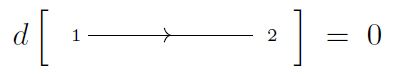###### Example

(differential on linear graphs)

The differential of Def. applied to the linear graph with one internal vertex: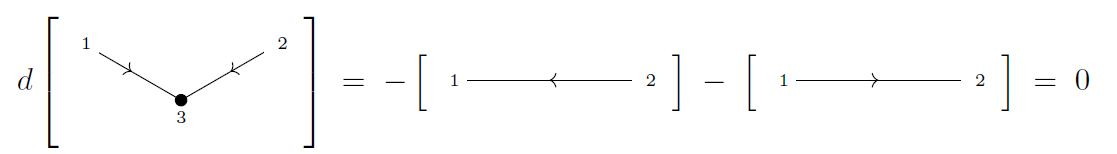Here the right hand side vanishes, due to the sign rule (7).

While this example illustrates the general action of the differential, beware that this particular differential relation will not actually contribute to the graph complex, as the graph on the left is a “vanishing graph” in the sense of Def. below (since the valence of the internal vertex is $\lt 3$).

###### Example

(differential of trivalent diagram with single internal vertex)

The image of the single trivalent internal vertex under the differential from Def. is as shown in the following: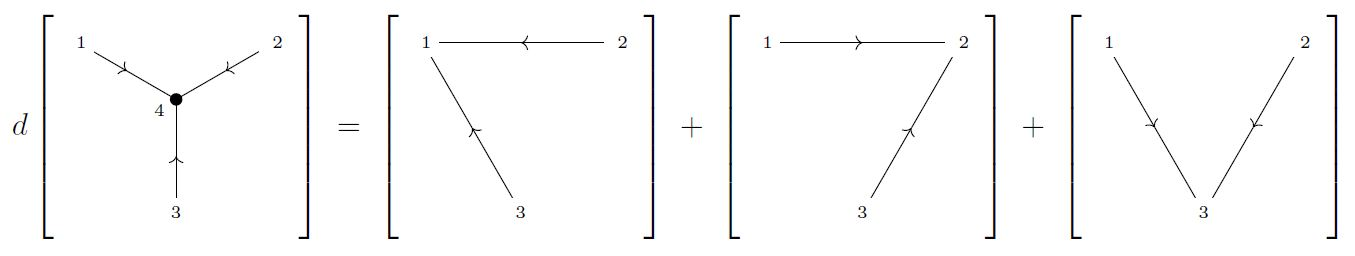Under the quasi-isomorphism (1) from the graph complex to the de Rham complex on the Fulton-MacPherson compactification of a configuration space of points, given by sending each graph to its Chern-Simons Feynman amplitude on compactified configuration spaces of points (this Prop.), this relation becomes the “3-term relation” (this Prop.):

$\left[g_{i j}\right] \wedge \left[ g_{j k} \right] + \left[g_{j k}\right] \wedge \left[ g_{k i} \right] + \left[g_{k i}\right] \wedge \left[ g_{i j} \right] \;\sim\; 0$

satisfied by the Chern-Simons propagator form

$\left( g_{i j} \;\in\; \Omega^2_{PA} \Big( \underset{{}^{1,\cdots,n}}{Conf} \big( \mathbb{R}^D \big) \Big) \right) \,.$
###### Example

(Differential of minimal trivalent vacuum diagram)

The differential of the minimum trivalent vacuum diagram from Example :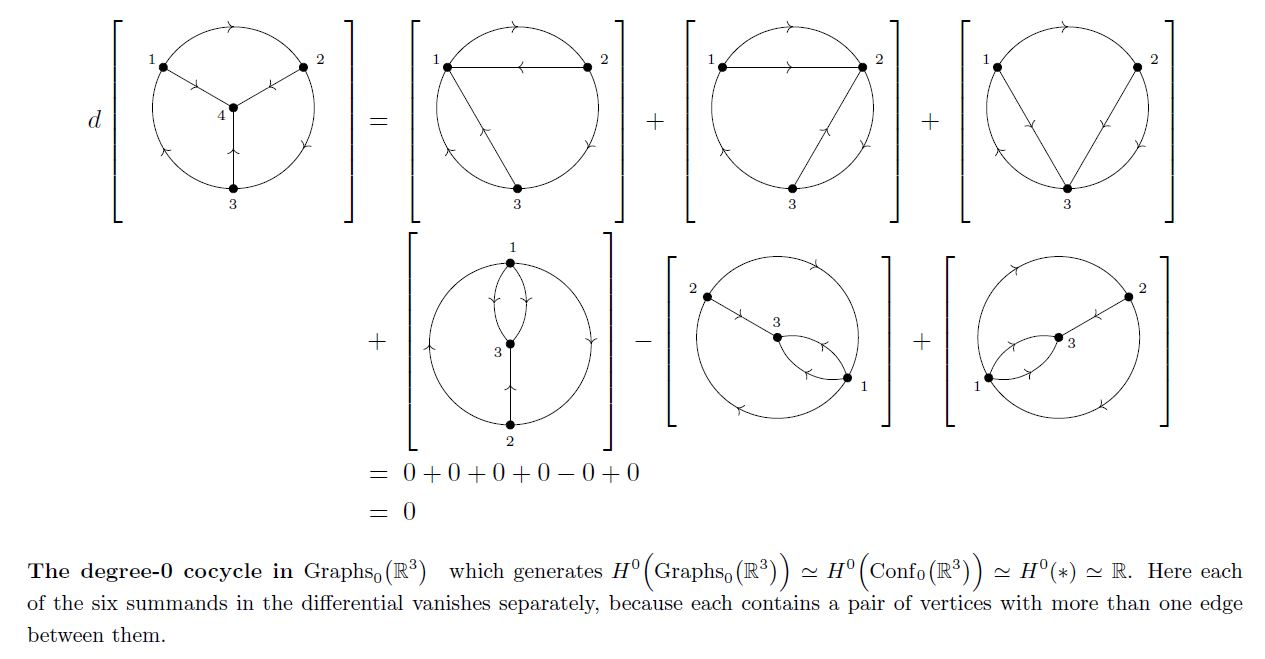###### Example

(differential of trivalent tree with two internal vertices)

The differential (Def. ) on the trivalent tree with two internal vertices: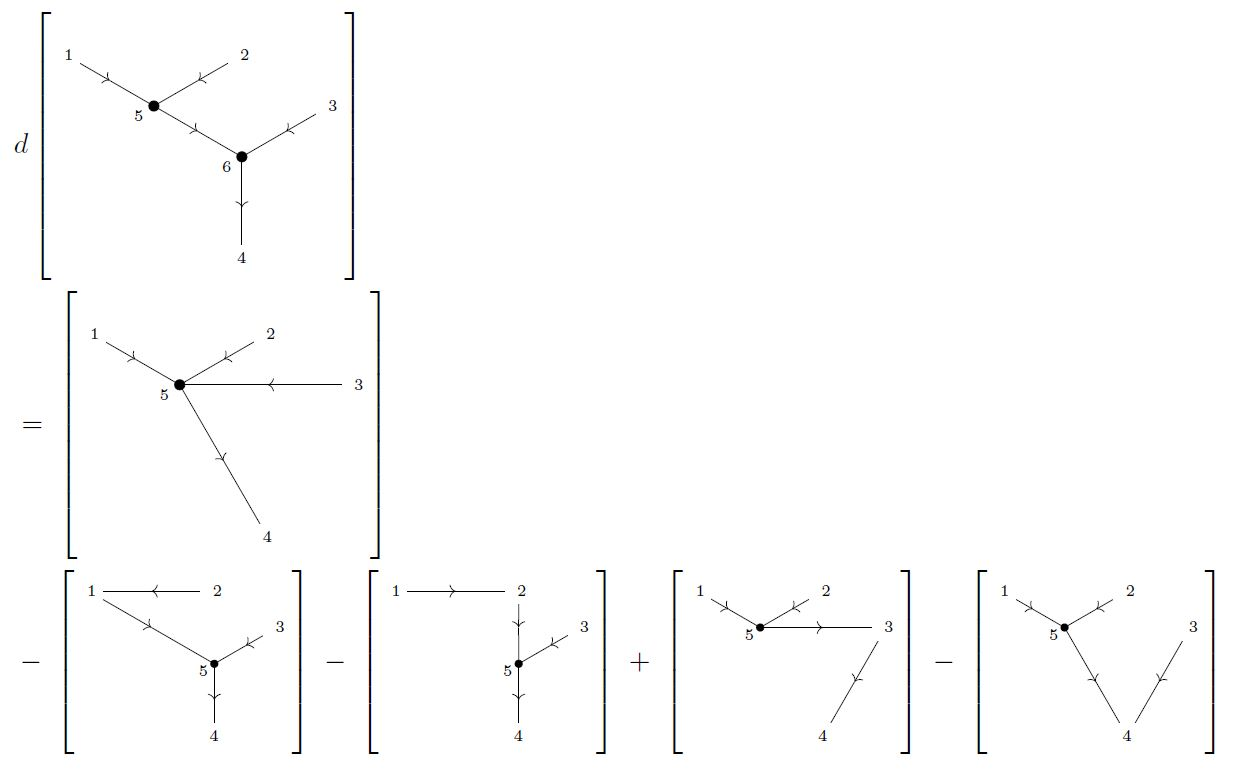###### Lemma

The linear map $d$ in Def. makes the graded vector space $\widehat Graphs_n(\mathbb{R}^D)$ a cochain complex, and, with its graded algebra-structure from Lemma in fact a differential graded algebra, in that:

1. (well defined) $d$ indeed only depends on the equivalence class $[\Gamma]$;

2. (degree) $d$ increases degree by +1

3. (nilpotency)

$d \circ d = 0$
4. $d( [\Gamma_1] \wedge [\Gamma_2] ) \;=\; \big( d[\Gamma_1] \big) \wedge [\Gamma_2] + (-1)^{deg(\Gamma_1)} [\Gamma_1] \wedge \big( d [\Gamma_2]\big) \,.$

##### The graph complex
###### Definition

(vanishing graphs)

We say that a graph $\Gamma$ (Def. ) is a vanishing graph if it is one or more of the following:

• it is a tadpole-diagram: $\Gamma$ contains an edge $e$ with $s(e) = t(e)$ (Example );

• it is a vacuum diagram: $\Gamma$ contains an internal vertex which is not connected, via some path of edges, to an external vertex;

• it has parallel edges: $\Gamma$ contains a pair $(a,b)$ of vertices, with more than one edge between them, i.e. if the preimage $(s,t)^{-1}\big\{(a,b)\big\}$ contains more than one element.

• it is less than trivalent: $\Gamma$ contains an internal vertex $v \in Verts_{int}$ that is less than trivalent, hence an internal vertex with less than 3 edges attached to it;

Write

${\widehat Graphs}^{nil}_n(\mathbb{R}^3) \;\subset\; \widehat Graphs_n\big( \mathbb{R}^3 \big)$

for the subspace spanned by vanishing graphs $[\Gamma]$ inside the graded vector space (9) of all graphs.

###### Lemma

(vanishing graphs form a differential ideal)

The subspace of vanishing graphs (Def. ) is a differential ideal in the differential graded-commutative algebra of all graphs (Lemma ).

This implises that the quotient of all graphs by the vanishing graphs is still a differential graded-commutative algebra:

###### Definition

(graph complex)

For $n \in \mathbb{N}$, the graph complex on $n$ external vertices is the differential graded-commutative algebra

(12)$Graphs_n \big( \mathbb{R}^3 \big) \;\coloneqq\; \frac{ {\widehat Graphs}_n\big( \mathbb{R}^3\big) } { {\widehat Graphs}^{nil}_n\big( \mathbb{R}^3\big) }$

of the differential graded-commutative algebra of all graphs (Lemma ) by its differential ideal (Lemma ) of vanishing graphs (Def. ).

###### Example

(A 9-cocycle in $Graphs_3(\mathbb{R}^3)$)

The trivalent graph of degree 9 from Example is a cocycle in $Graphs_3(\mathbb{R}^3)$ (Def. ):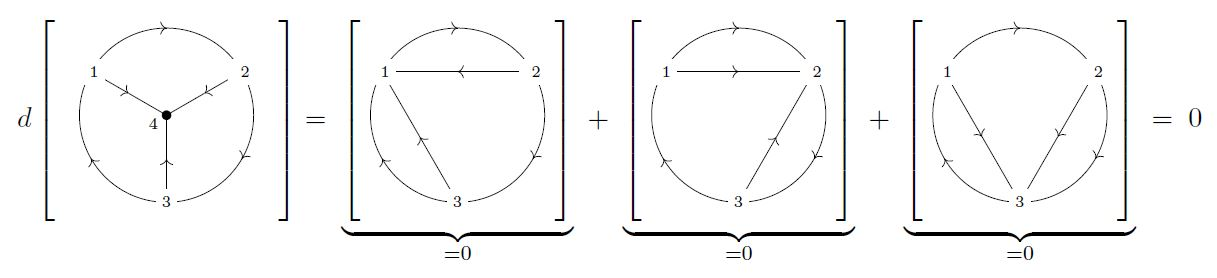The computation of the differential is just as for the 3-term relation in Example , but now all summands on the right have parallel edges and hence are vanishing graphs (Def. ) that are zero in the quotient (12) defining the graph complex $Graphs_3\big( \mathbb{R}^3 \big)$ (Def. ).

This trivalent diagram must be exact, as there is not supposed to be any cohomology in degree 9 (by Prop. ). What’s a trivializing coboundary?

###### Lemma

(graphs are in non-negative degree)

For all $n \in \mathbb{N}$, the degrees (Def. ) of any non-vanishing graph $[\Gamma]$ in the graph complex $Graphs_n(\mathbb{R}^3)$ (Def. ) are non-negative

$deg\big( [\Gamma] \big) \;\geq\; 0 \;\; \in \;\; Graphs_n(\mathbb{R}^3) \,.$

Moreover, the only graphs of degree 0 are those containg the external vertices and nothing else:

$deg\big( [\Gamma]\big) = 0 \;\;\;\;\;\;\Leftrightarrow\;\;\;\;\;\; [\Gamma] = 0 \;\;\;\text{or}\;\;\; [\Gamma] = \big[ {}_1 \;\; {}_2 \;\; \cdots \;\; {}_n \big] \;\;\;\;\in\;\; Graphs_n(\mathbb{R}^3) \,.$
###### Proof

Since tadpole graphs are vanishing graphs by Def. , we may assume that each edge in $\Gamma$ has two distint vertices it ends on, for otherwise we have the zero element in the graph complex.

Now, since each edge has degree 2 (according to Def. ), we may think of each edge as contribution a degree +1 for each of its two vertices, via the coresponding half-edge ending on that vertex.

Since external vertices have degree 0, together with the half-edges that end on them they contribute a non-negative number to the total degree.

While internal vertices have degree -3 (according to Def. ), we have a vanishing graph if less than three edges meet at an internal vertex (by Def. ) and hence, once vanishing graphs have been quotiented out, also internal vertices together with the half-edges ending on them contribute a non-negative number to the total degree.

This proves the first claim.

For the second claim, recall from Example that a graph has degree 0 precisely if it is a disjoint union of a) isolated external vertices and b) trivalent graphs all whose vertices are internal.

But if there is any disjoint non-empty sub-graph with only internal vertices, the total graph is again a vanishing graph by Def. , since it contains internal vertices not connected to any external vertex.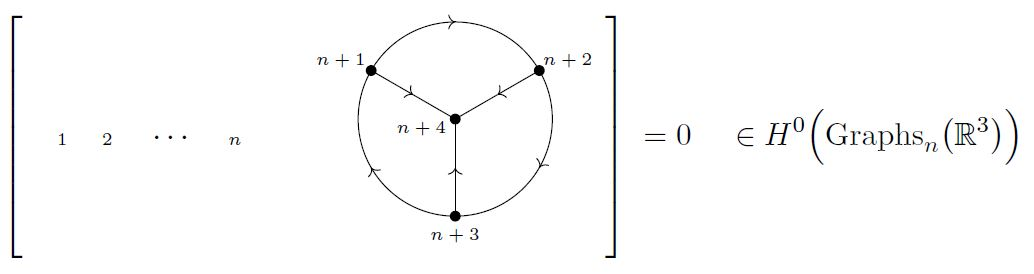Hence the only non-vanishing graphs of degree 0 are those which have some isolated external vertices and nothing else.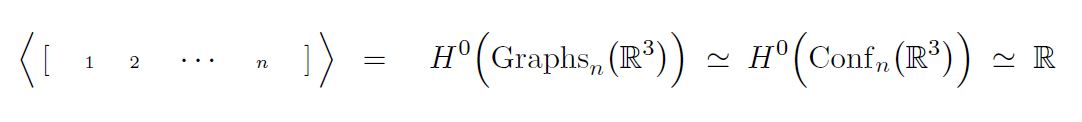(…)

### 2) Graph complexes modelling spaces of knots

We discuss graph complexes $KnotGraphs(\Sigma)$ modelling the real cohomology of spaces of knots $Emb(S^1,\Sigma)$.

For the case of $\Sigma = \mathbb{R}^D$ these were originally hinted at in Kontsevich 93, Section 5 and discussed in detail in Cattaneo, Cotta-Ramusino, Longoni 02 05, see also Bar-Natan 91. Review is in Volić 13, Section 4.

#### $KnotGraphs\big( \mathbb{R}^3 \big)$

We state the definition of the graph complexes $KnotGraphs\big( \mathbb{R}^3\big)$ of knot graphs (according to the above) associated with Euclidean space of dimension 3, following Cattaneo, Cotta-Ramusino, Longoni 02 05.

The definition is almost exactly the same as that of $\oplus_n Graphs_n\big( \mathbb{R}^3\big)$ above, except for the following two differences:

1) external vertices now carry degree -1, so that the formula (3) for the degree of a graph is changed to

(13)$deg\big( [\Gamma]\big) \;=\; (D-1) \cdot \#\!Edges \; - D \cdot \#\!Vertices_{int} \; - 1 \cdot \#\!Vertices_{ext} \,,$

2) There is implicit a contractible edge from the $k$th to the $(k+1)$st external vertex (an arc), in that the definition of the differential (Def. ) regards these as contractible edges.

3) Apparently it must be understood that graphs whose labelling of external vertices differs by a cyclic permutation are identified. (?!)

(…)

###### Example

(knot graph cocycle of order 2)

The differential in $KnotGraphs(\mathbb{R}^3)$ of the single trivalent internal vertext is a variant of that in $Graphs_3\big(\mathbb{R}^3\big)$ (Example ) and that in $Graphs_0\big( \mathbb{R}^3\big)$ (Example ):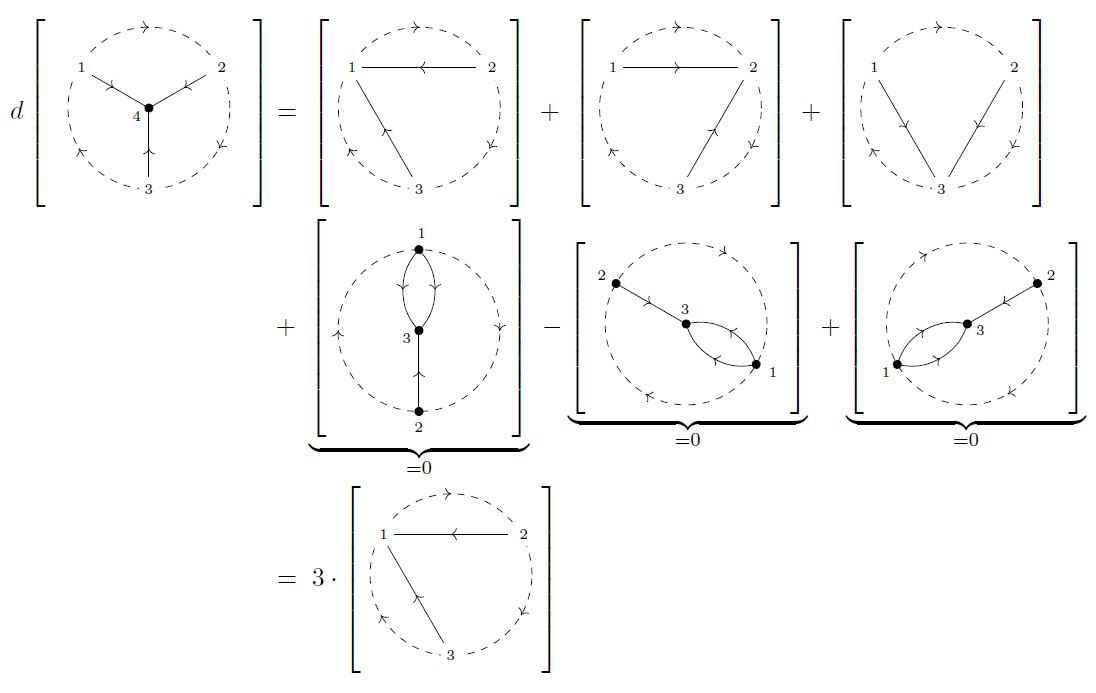Notice how the edges parallel to the outer dashed arcs do not make the graphs in the first line vanish, but the parallel edges appearing in the second line make vanishing graphs (Def. ).

In contrast, the differential of the diagram in $KnotGraphs(\mathbb{R}^3)$ consisting of just two overlapping chords gets contributions only from contraction of the four arcs: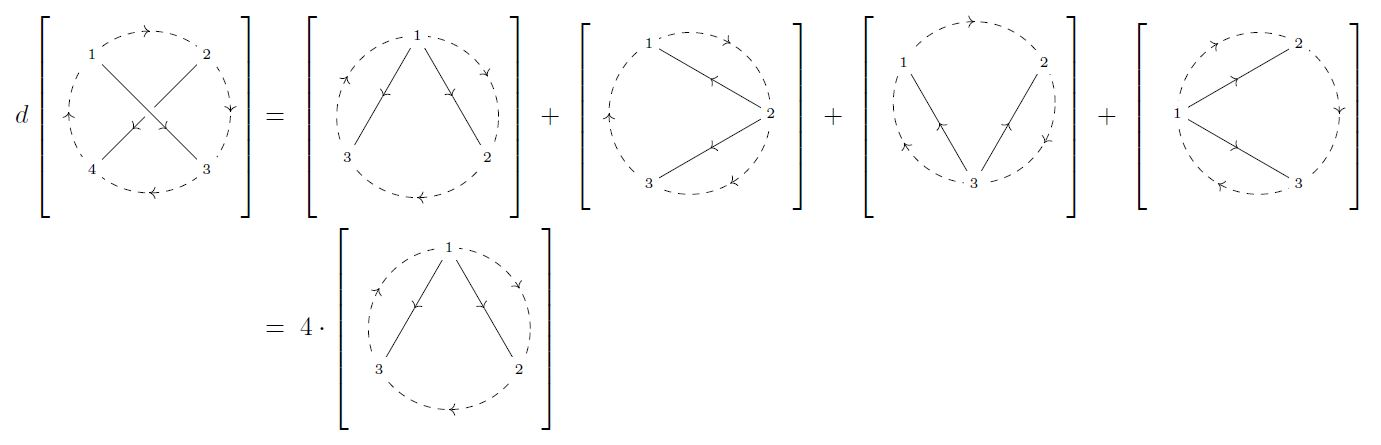In both cases, the identification of graphs whose external labels are ccyclically permutated identifies the right hand sides of both these coboundaries with multiples of one and the same element in the graph complex.

Accordingly, the corresponding linear combination

is a cocycle of degree 0 in $KnotGraphs(\mathbb{R}^3)$: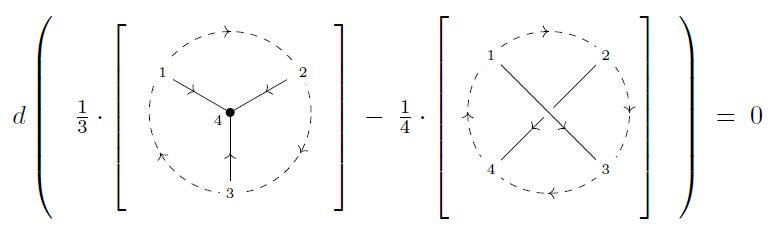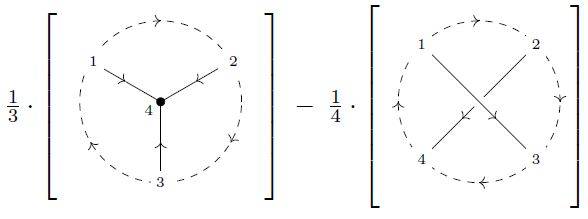$\in H^0\Big( KnotGraphs\big( \mathbb{R}^3 \big) \Big)$

For the corresponding Feynman amplitude knot invariant see Prop. below.

## Properties

under construction

### Cohomology of configuration spaces via Feynman diagrams

Reading the graphs as Chern-Simons theory Feynman diagrams, hence as instructions for configuration space-integrals of wedge products of Feynman propagators assigned to edges in a graph, with propagators regarded as differential forms on configuration spaces, yields a quasi-isomorphism from the graph complex to the real cohomology of configuration spaces of points.

$\underset{ \color{blue} \array{ \phantom{A} \\ \text{graph complex} \\ \text{of n-point Feynman diagrams} \\ \text{for Chern-Simons theory} \\ \text{on} \; \Sigma } }{ Graphs_n(\Sigma) } \underoverset{ \simeq_{\mathrlap{qi}} } { \color{blue} \array{ \text{assign Feynman amplitudes} \\ \text{of Chern-Simons theory} \\ \text{by configuration-space integral} \\ \text{of internal vertices over}\; \Sigma \\ \phantom{A} } } { \longrightarrow } \underset{ \color{blue} \array{ \phantom{A} \\ \text{de Rham algebra} \\ \text{of semi-algebraic differential forms} \\ \text{on the FM-compactification} \\ \text{of the configuration space of n points} \\ \text{in}\; \Sigma } }{ \Omega^\bullet_{PA} \big( \underset{{}^{1,\cdots,n}}{Conf}\big( \Sigma \big) \big) } \,.$
###### Proposition

(real cohomology of configuration spaces of ordered points in Euclidean space)

The real cohomology ring of the configuration spaces

$\underset{{}^{1,\cdots,n}}{Conf}\big( \mathbb{R}^D \big) \;\coloneqq\; \big( \mathbb{R}^D \big)^n \setminus FatDiag$

of $n$ ordered points in Euclidean space $\mathbb{R}^D$ is generated by elements

$\omega_{i j} \;\; \in H^2 \Big( \underset{{}^{1,\cdots,n}}{Conf}\big( \mathbb{R}^D \big), \mathbb{R} \Big)$

for $i, j \in \{1, \cdots, n\}$

subject to these three relations:

1. $\omega_{i j} = (-1)^D \omega_{j i}$;

2. $\omega_{i j} \wedge \omega_{i j} \;=\; 0$;

3. $\omega_{i j} \wedge \omega_{j k} + \omega_{j k} \omega_{k i} + \omega_{k i} \wedge \omega_{i j} = 0$.

Hence:

$H^\bullet \Big( \underset{{}^{1,\cdots,n}}{Conf}\big( \mathbb{R}^D \big), \mathbb{R} \Big) \;\simeq\; \mathbb{R}\Big[ \big\{\omega_{i j} \big\}_{i, j \in \{1, \cdots, n\}} \Big] \Big/ \left( \array{ \omega_{i j} = (-1)^D \omega_{j i} \\ \omega_{i j} \wedge \omega_{i j} = 0 \\ \omega_{i j} \wedge \omega_{j k} + \omega_{j k} \omega_{k i} + \omega_{k i} \wedge \omega_{i j} = 0 } \;\; \text{for}\; i,j \in \{1, \cdots, n\} \right)$

This is due to Arnold 69, Cohen 73.

real cohomology of configuration space of pointsgraph complex (this Prop.)
generator
$\omega_{i j} \in H^2\Big( \underset{{}^{1,\cdots,n}}{Conf}\big( \mathbb{R}^3\big) \Big)$
edge
$i \longrightarrow j$
relations:relations:
$\omega_{i j} = - \omega_{j i}$graph changes sign when edge is reversed (Def. )
$\omega_{i j} \wedge \omega_{i j} \;=\; 0$graph with parallel edges vanishes (Def. )
$\omega_{i j} \wedge \omega_{j k} + \omega_{j k} \omega_{k i} + \omega_{k i} \wedge \omega_{i j} = 0$coboundary of trivalent vertex (Example )

### Cohomology of knot space / higher Vassiliev invariants

The Feynman amplitudes of knot graphs (as above) are Vassiliev knot invariants.

This was originally hinted at in Kontsevich 93, Section 5. Details are in Cattaneo, Cotta-Ramusino, Longoni 02. Review is in Volić 13, Section 4.

$\underset{ \color{blue} \array{ \phantom{A} \\ \text{graph complex} \\ \text{of n-point Feynman diagrams} \\ \text{for Chern-Simons theory} \\ \text{on} \; \Sigma } }{ KnotGraphs(\Sigma) } \underoverset{ } { \color{blue} \array{ \text{assign Feynman amplitudes} \\ \text{of Chern-Simons theory} \\ \text{by configuration-space integral} \\ \text{of internal vertices over}\; \Sigma \\ \text{and of external vertices along the knot} \\ \phantom{A} } } { \longrightarrow } \underset{ \color{blue} \array{ \phantom{A} \\ \text{de Rham algebra of} \\ \text{space of knots in}\; \Sigma } }{ \Omega^\bullet_{PA} \big( Emb\big( S^1, \Sigma\big) \big) } \,.$
###### Proposition

(knot invariant from Chern-Simons theory Feynman amplitude)

The Feynman amplitude of the knot graph cocycle Feynman diagram from Example , in Chern-Simons theory with a Wilson loop knot, is a knot invariant: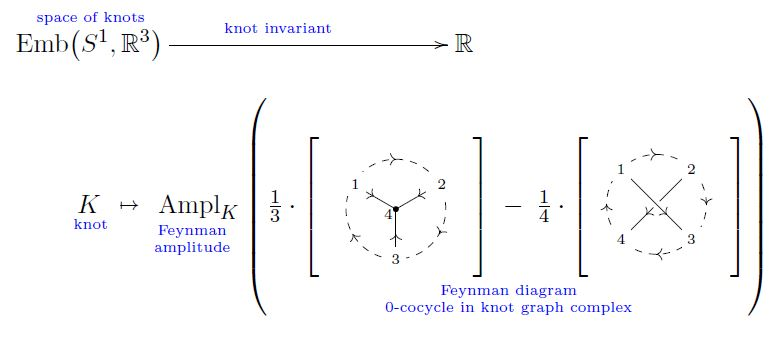This is due to Guadagnini-Martellini-Mintchev 89 and Bar-Natan91, recalled im Volić 13, Theorem 3.3.5.

Various authors discuss Vassiliev invariants in terms of graphs subject to the “STU-relation

(Kontsevich 93, Figure 8, Bar-Natan 95, Figure 3) and the “IHX-relation” which it implies (Bar-Natan 95, Theorem 6)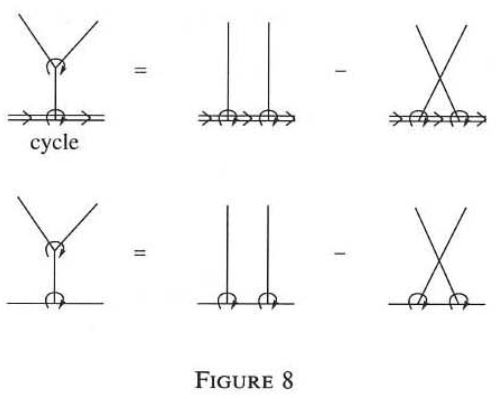graphics grabbed from Kontsevich 93

That these relations characterize the cohomology of the knot-graph complex in the respective degrees is shown in Koytcheff-Munson-Volic 13, Section 3.4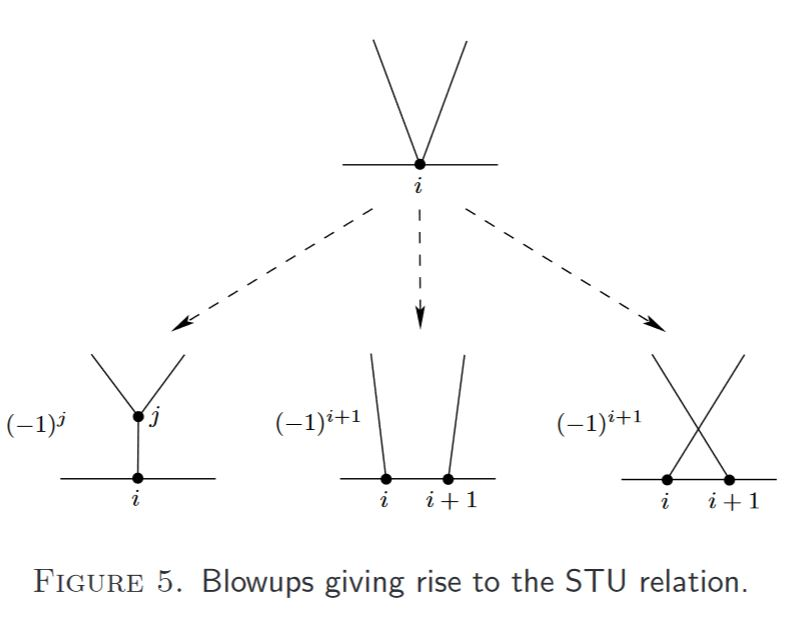graphics grabbed from Koytcheff-Munson-Volic 13

### Cohomology of knot graph complex is weight systems on chord diagrams

cohomology of knot graph complex is weight systems on chord diagrams

## Further applications

…moduli spaces

…deformation theory

…Rozansky-Witten theory

…description of the classifying space $BOut(F_n)$ of the group of outer automorphisms of a free group with $n$ generators

… another graph complex controls the universal $L_\infty$-deformations of the space of polyvector fields.

### General

The rough definition of the graph complex, and its relation to Chern-Simons theory Feynman amplitudes and Vassiliev knot invariants was sketched in

• Maxim Kontsevich, pages 11-12 of Feynman diagrams and low-dimensional topology, First European Congress of Mathematics, 1992, Paris, vol. II, Progress in Mathematics 120, Birkhäuser (1994), 97–121 (pdf)

• Maxim Kontsevich, Section 5 of: Vassiliev’s knot invariants, Advances in Soviet Mathematics, Volume 16, Part 2, 1993 (pdf, pdf)

• Maxim Kontsevich, around Def. 15 and Lemma 3 in Operads and Motives in Deformation Quantization, Lett. Math. Phys. 48 35-72, 1999 (arXiv:math/9904055)

### As a rational model for configuration spaces

A clean account and proof of the graph complex as a model for the rational homotopy type of the Fulton-MacPherson compactification of configuration spaces of points (exhibiting the formality of the little n-disk operads) is in

Discussion as a direct model for the rationalized homotopy groups of the configuration space of points:

further review:

Further discussion of the graph complex as a model for the de Rham cohomology of configuration spaces of points is in

The following survey has discussion of context between the graph complex and Batalin-Vilkovisky formalism:

### As construction of higher order Vassiliev invariants

Discussion of the graph complex as computing higher order Vassiliev invariants, hence the real cohomology of spaces of knots (with the cohomology in degree-0 being the ordinary Vassiliev invariants):

Reviewed in:

### Coproduct

Discussion of coproducts on a graph complex, given by decomposition of graphs into subgraphs and contractions of subgraphs:

### Cohomology

On the cochain cohomology of graph complexes:

Characterization of cohomology of (…) in terms of STU-relations and HKX-relations:

• Robin Koytcheff, Brian A. Munson, Ismar Volić, Section 3.4 of: Configuration space integrals and the cohomology of the space of homotopy string links, J. Knot Theory Ramif. 22, no. 11, 73 pp. (2013) (arXiv:1109.0056)

Concrete examples of cohomology classes in certain bi-degrees in $Graphs_0(\mathbb{R}^2)$ by computer experiment:

$H^0\big( Graphs_0(\mathbb{R}^2) \big)$ is the Lie algebra of the Grothendieck-Teichmüller group: